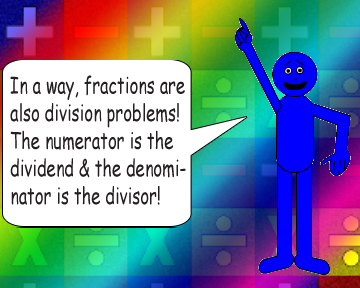# The Names of Numbers in Math Problems## A is the base, B is the exponent & C is the power.

### Note: When B is equal to a non-integer, consider radicals! Why? Because raising a number to the power of a non-integer, like ½, is the same as calculating the square root! It depends on what the numerator is or what the denominator is...### Okay, I'll give you a little hint about it: